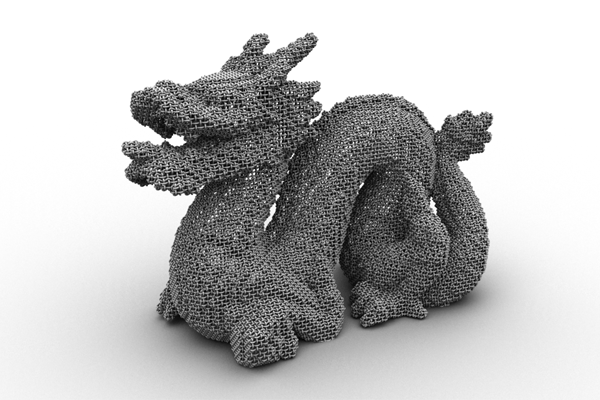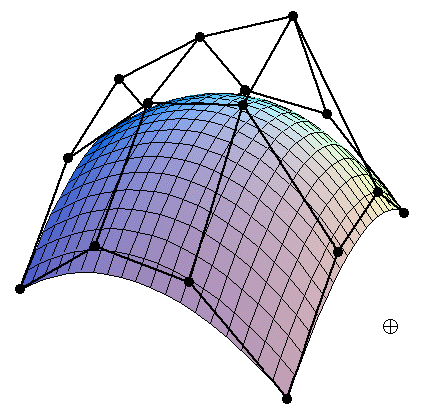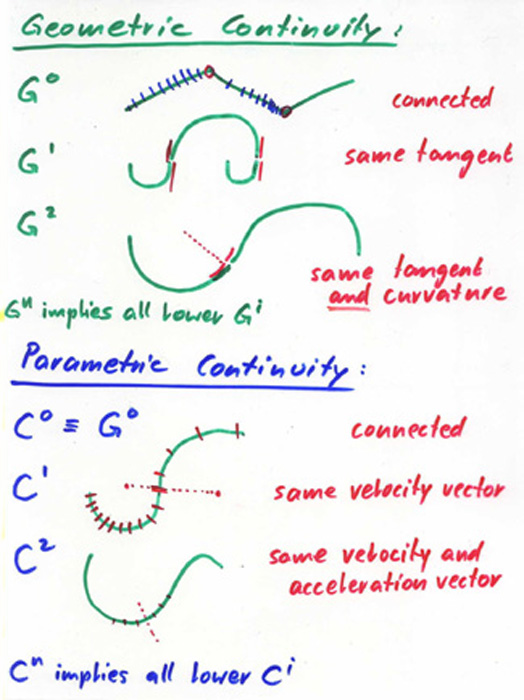# CS184 Section 7 Bezier Curves and De Casteljau's Algorithm

Brandon Wang
March 12, 2013
Material adapted from Fu-Chung Huang.

### Today

Midterm next week!

• Curves: Why?
• Curves Worksheet
• C, G continuity (maybe)

### Curves

In the end, you'll be sending triangles to your GPU to render, so why represent things in this weird format?

• Scale/arbitrary precision (fonts, vector graphics)
• Some objects are easier to represent in this way
• The sampling algorithm isn't too bad.

### Curves?

Smooth curves. Triangles are jagged, etc. Think of it as a continuous representation of a curve, whereas triangles are a discrete sample. (Demo later)

### Nice Curves### Geri's Game

http://youtu.be/1m7dcbIKvlw

### De Casteljau's Algorithm

Calculate f(u) at a specific point u. (Easier to think between 0 and 1)

Split each line at u.### De Casteljau Animation### De Casteljau Animation(Oh man Wikipedia is awesome)

### Finite Subdivision

Can't calculate f(u) for all u. That's silly.

Compute a few points, then draw lines between them.

AS3 demo

### Pseudocode

Dynamic Programming

```for i = 0:power
p[i][power] = control_points[i]
for power = degree-1:0
for i = 0:power
p[i][power] = (1-u) * p[i][power+1] + u*p[i+1][power+1]
return p
```

### Bezier SurfacesWorksheet

### Geometric, Parametric Continuity